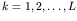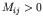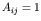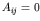Computational & Technology Resources an online resource for computational,engineering & technology publications not logged in - login Civil-Comp ProceedingsISSN 1759-3433 CCP: 84PROCEEDINGS OF THE FIFTH INTERNATIONAL CONFERENCE ON ENGINEERING COMPUTATIONAL TECHNOLOGY Edited by: B.H.V. Topping, G. Montero and R. Montenegro Paper 223A New Algorithm for Detecting Graph Symmetry for Use in Engineering Problems M. SalariResearch Centre of Building and Housing, Tehran, Iran doi:10.4203/ccp.84.223 Full Bibliographic Reference for this paper M. Salari, "A New Algorithm for Detecting Graph Symmetry for Use in Engineering Problems", in B.H.V. Topping, G. Montero, R. Montenegro, (Editors), "Proceedings of the Fifth International Conference on Engineering Computational Technology", Civil-Comp Press, Stirlingshire, UK, Paper 223, 2006. doi:10.4203/ccp.84.223 Keywords: symmetry, algorithm, graph, axis of symmetry, computation, eigenvalue. Summary Symmetry has been widely applied to many problems in engineering. Usually, one can correspond a graph to an engineering model. If this graph is symmetric, one can use this feature for more efficient solution of the problem. For using such a benefit, graph symmetry must be first detected. Several algorithms exist for detecting graph symmetry. They are suitable for most graphs; however they fail for some others. In this paper a new algorithm is proposed for detecting graph symmetry. As a new feature, this algorithm tests a graph for symmetry and reveals if the graph is symmetric or not. Moreover, the algorithm finds all symmetries such as axial and central symmetry without neglecting any of them. Besides, the proposed algorithm with inherent robustness does not fail for any graph types. Therefore, there is no restriction on using this algorithm. The method used in this algorithm is based on studying the graph theoretical properties of nodes of the graph. These properties are considered as the degree of the nodes, patterns of the shortest root tree (SRT) rooted from the nodes, degree of adjacent nodes, and so on. In this method we search for nodes that have the same properties. By a particular search we find corresponding nodes and the nodes and members on the axis of symmetry. In order to study the pattern of the SRT rooted from the nodes, the kth neighbour of a node is defined; if the minimum distance of two nodes in the graph is k, each of them is the kth neighbour of the other. So the length of SRT rooted from the nodes, the number of the kth neighbour of the nodes, and the degree of kth neighbour(s) forare taken as a part of SRT rooted from the nodes (L is the length of the graph). In order for two nodes to be corresponding, they must be located in the same situation with regard to the axis of symmetry and have the same graph-theoretical properties. The basis for the algorithm introduced is the comparison of the properties of the nodes in a graph. The comparison could be accomplished in different ways. The seemingly most straightforward way is a comparison of all the nodes, which is very time-consuming. Here a shorter way is set forth. First two corresponding nodes of the graph are found and taken as a benchmark for determining the nodes and members on the axis of symmetry of the graph. Then we remove the members and the nodes that are located on the axis of symmetry and the members whose one end is located on the axis of symmetry. So the graph is converted into two subgraphs the nodes of which are compared. If the graph is symmetric, the two subgraphs will be isomorphic. For detecting all symmetries, a set of nodes having the least length of the STR rooted from them are considered and all of the corresponding nodes in this set are found. Selecting each member of the afore-mentioned set leads us to another possibly new axis of symmetry. Symmetry in an engineering system can be of various types. For example the model corresponding to an engineering system may have geometrical symmetry; but the possibility of the existence of such symmetry is low and in case this symmetry exists, it can be easily detected. On the other hand the model corresponding to system can be topologically symmetric. In this case the graph corresponding to the model would be symmetric. So this symmetry can be used for the efficient solution of engineering problems. Furthermore, suppose that an engineering problem lead to a mathematical calculation in a matrix M. Matrix A corresponding to M can be constructed in this manner: ifthen takeor else. The constructed matrix A actually is an adjacency matrix of a graph. Therefore if the graph is symmetric, we can use this feature for a more efficient solution of the problem. The detection of the topological symmetry of an engineering model or, in other words, the detection of the symmetry of the graph corresponding to an engineering model is very difficult. But the algorithm introduced can quickly detect the symmetry of a graph and find all the axis of symmetry. The speed of the algorithm is high enough and it decreases the number of comparisons. The algorithm does not fail for any graph types. This algorithm has been implemented using the TURBO C language and satisfactory results have been obtained. The speed of the algorithm depends on the number of the nodes of the graph and the length of the graph. References 1 A.Kaveh, "Structural Mechanics: Graph and Matrix Method", RSP (John Wiley), UK, 2004. purchase the full-text of this paper (price £20) Back to top ©Civil-Comp Limited 2020 - terms & conditions